### Home > PC3 > Chapter 8 > Lesson 8.1.3 > Problem8-52

8-52.
1. Write an equation for each curve below. State the equation of the parent function and describe the transformation. Homework Help ✎

1.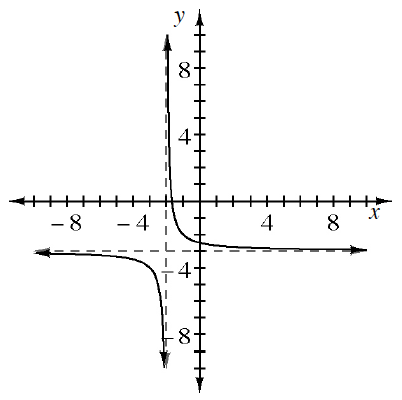2.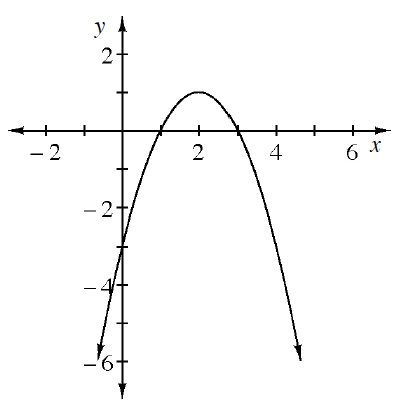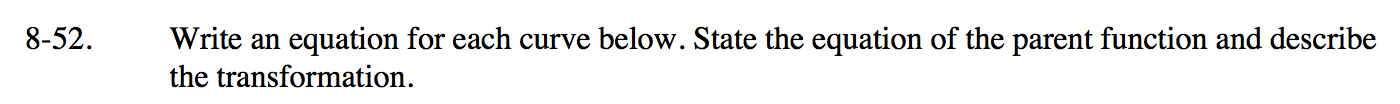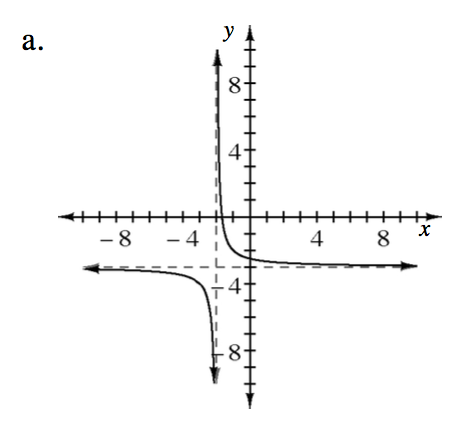$y=\frac{a}{x-h} +k$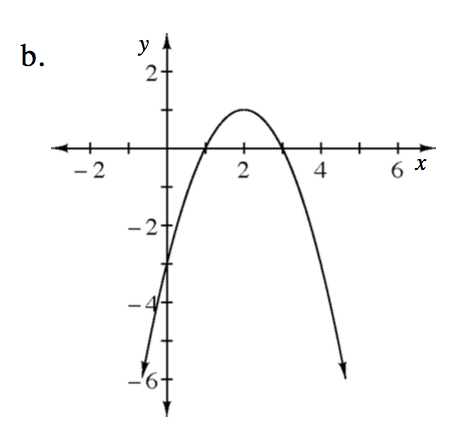$y = a(x-h)^2+k$